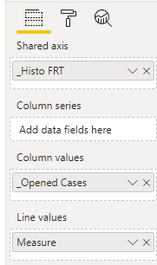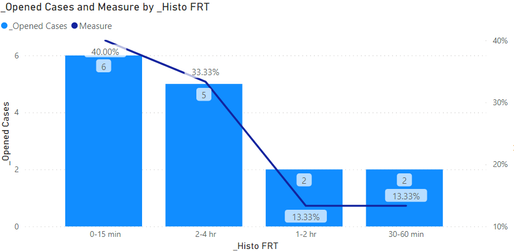cancel
Showing results for
Did you mean:Anonymous
Not applicable

## Cumulative Sum and Cumulative Sum Percentage

Hi,

I have a dataset which contains a case number, opended date, response target, response deviation in minutes. I added a custom column called First Response Calc for calculating the response target - response deviation in minutes.I created a column named  _First Response (hours) which calculates the date difference in hours because the Date/Time Opened and First Reponse Calc field:

_First Response (hours) = DATEDIFF('Sheet1'[Date/Time Opened],'Sheet1'[First Response Calc], MINUTE) /60

I created an additional column called _Histo FRT which breaks down the First Response Hours based on time frames:

_Histo FRT = IF('Sheet1'[_First Response (hours)] < 0.25, "0-15 min",IF('Sheet1'[_First Response (hours)] < 0.5, "15-30 min", IF('Sheet1'[_First Response (hours)] < 1, "30-60 min", IF('Sheet1'[_First Response (hours)] < 2, "1-2 hr", IF('Sheet1'[_First Response (hours)] < 4 , "2-4 hr", IF('Sheet1'[_First Response (hours)] < 8, "4-8 hr", IF('Sheet1'[_First Response (hours)] < 12, "8-12 hr", IF('Sheet1'[_First Response (hours)] < 24, "12-24", IF('Sheet1'[_First Response (hours)] < 36, "24-36 hr", IF('Sheet1'[_First Response (hours)] < 48, "36-48 hr", IF('Sheet1'[_First Response (hours)] < 72, "2-3 days", IF('Sheet1'[_First Response (hours)] < 120, "3-5 days", IF('Sheet1'[_First Response (hours)] < 240, "5-10 days", If('Sheet1'[_First Response (hours)] < 480, "10-20 days"))))))))))))))

_Opened Cases is a column that calculates the distinct count of Case Numbers

_Opened Cases = CALCULATE(DISTINCTCOUNT('Sheet1'[CaseNumber]))

When I create a Line and Stacked Column chart I have the _History FRT on the X-axis and the _Open Cases on the Y-axis. This shows the number of Open Cases per History grouping as such (and works good).What I would like to do is show a line value of cumulative open case percentages. For example 359 for 0-15 minutes would be 32% of the entire chart for the date range selected. Right now my line chart is only showing open case amounts for each time frame just as the bar chart is.

How do I calculate the cumulative amount and cumulative percentage?

Thanks,

Kevin

1 ACCEPTED SOLUTIONCommunity Support

Hi  @Anonymous ,

Modify the measure to the following form:

1. Create measure.

``````Measure =
var _1=COUNTX(FILTER(ALL('Sheet1'),'Sheet1'[_Histo FRT]<=MAX('Sheet1'[_Histo FRT])),[CaseNumber])
var _2=COUNTX(ALL('Sheet1'),'Sheet1'[_Opened Cases])
return
DIVIDE(_1,_2)``````

2. Result:Best Regards,

Liu Yang

If this post helps, then please consider Accept it as the solution to help the other members find it more quickly

6 REPLIES 6Community Support

Hi  @Anonymous ,

Modify the measure to the following form:

1. Create measure.

``````Measure =
var _1=COUNTX(FILTER(ALL('Sheet1'),'Sheet1'[_Histo FRT]<=MAX('Sheet1'[_Histo FRT])),[CaseNumber])
var _2=COUNTX(ALL('Sheet1'),'Sheet1'[_Opened Cases])
return
DIVIDE(_1,_2)``````

2. Result:Best Regards,

Liu Yang

If this post helps, then please consider Accept it as the solution to help the other members find it more quicklyAnonymous
Not applicable

This works great! Thanks for the help.Community Support

Hi  @Anonymous ,

Here are the steps you can follow：

1. Create measure.

``````Measure =
var _1=COUNTX(FILTER(ALL('Sheet1'),'Sheet1'[_Histo FRT]=MAX('Sheet1'[_Histo FRT])),[CaseNumber])
var _2=COUNTX(ALL('Sheet1'),'Sheet1'[_Opened Cases])
return
DIVIDE(_1,_2)``````

2. Place [Measure] in Line values.3. Result:Best Regards,

Liu Yang

If this post helps, then please consider Accept it as the solution to help the other members find it more quicklyAnonymous
Not applicable

Hi Liu,

The calculation you provided calculates the percentage of the total for each bar correctly (thank you).

I am looking to see a culmulative percentage though. If the first bar is 40%, then the second bar which is 33% should be 73.33% since it's a culumative percentage.

The bars and percentages should show:

Bar 1: 40%

Bar 2: 73.33%

Bar 3: 86.66

Bar 4: 100%

Is this something that can be done?

Thanks,

KevinSuper User

Hello there @Anonymous ! Just as a suggestion, to simplify your coding steps and the readability the formulas you develop try using the SWITCH function instead of the multiple IFs() in the  "_Histo FRT". It may not only help you create simpler measures but also evaluate possible errors.

Regarding your question, you can check similar these threads with solutions provided for similar questions to yours:

https://community.powerbi.com/t5/Desktop/cumulative-running-total-percentage/m-p/384190

https://community.powerbi.com/t5/Desktop/Calculate-cumulative-percentage-from-a-calculated-column/m-p/428926

https://community.powerbi.com/t5/Desktop/cumulative-percentages/m-p/571128

If my reply provided you with a solution, please consider marking it as a solution ✔️ or giving it a kudoe 👍
Thanks!

You can also check out my LinkedIn!

Best regards,
Gonçalo GeraldesAnonymous
Not applicable

Hi Goncalo,

Thanks for your reply. I looked into those three forum questions before I sent the question out. They don't seem to work for what I want to do. I don't have a product with a sales dollar amount.

I have a number of cases within a time range. I am looking to calculate the cumulative percentage of cases as it refers to a particular time instance (0-15 minutes, 30-60 minutes, etc..)